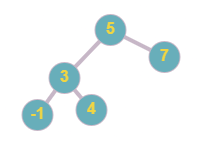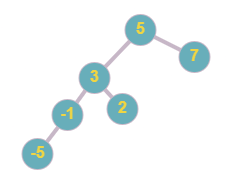# Convert Sorted Array to BST

Posted: 20 Mar, 2021
Difficulty: Easy

## PROBLEM STATEMENT

#### Note:

``````1. A balanced binary tree is a binary tree structure in which the left and right subtrees of every node differ in height by no more than 1.

2. A binary search tree is a binary tree data structure, with the following properties
a. The left subtree of any node contains nodes with value less than the node’s value.
b. The right subtree of any node contains nodes with values equal to or greater than the node’s value.
c. Right, and left subtrees are also binary search trees.
``````

#### Example:

``````Below tree, is a balanced binary search tree
````````````Below tree is not a balanced tree as the height of the left subtree and right subtree of node ‘5’ differs by more than 1. Moreover, the tree is also not a BST as node ‘2’ is less than node ‘3’ but ‘2’ is the right child of ‘3’.
``````#### Input Format:

``````The first line contains an integer 'T' which denotes the number of test cases or queries to be run.

The first line of each test case contains an integer ‘N’ which denotes the number of elements in the array.

The second line of each test case contains ‘N’ space-separated integers denoting the elements of the array in strictly increasing order.
``````

#### Output Format:

``````For each test case, the output will be “1” if you have returned the correct answer, else it will be “0”.
``````

#### Note :

``````You do not need to input or print anything, and it has already been taken care of. Just implement the given function.
``````

#### Constraints:

``````1 <= T <= 5
1 <= N <= 3000
1 <= arr[i] <= 10 ^ 5

Where 'arr[i]’ is the value of i-th element of the given array
arr < arr < arr . . . < arr[N].

Time Limit: 1 sec
``````Approach 1

As the given array is sorted and we want a balanced tree such that every node has a right subtree with a greater value and a left subtree with a smaller value. We can think of making the middle element of the array root node, this will ensure that both left and right subtree have equal elements thus maintaining the balance of the tree. All elements left of middle elements are smaller and go into the left subtree of the root node. Elements on the right of middle elements are greater thus goes on the right subtree. And also for making the left subtree a ‘balanced BST’ we can further divide it into two halves, same for the right subtree. Recursively following the procedure overall nodes will result in a balanced binary search tree.

## Algorithm :

• Let’s say we need to find a balanced BST for ‘ARR[1:N]’, where ‘N’ is the size of array ‘ARR’.
• Base case - if ‘N == 1’ i.e. only one element is present, then make it a root node.
• Else, Declare a variable say ‘mid’ that stores the middle index of the array.
• The ‘ARR[mid]’ forms the root node of balanced BST
• For left subtree recursively call the function with the left part of array i.e ‘ARR[1: mid-1]’
• For the right subtree recursively call the function with the right part of the array i.e. ‘ARR[mid+1:N]’
• Add returned left subtree and right subtree to the left and right child, respectively of the root node.
• Return root node.